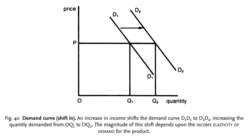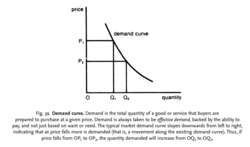# demand curve

Also found in: Wikipedia.

## demand curve

see EQUILIBRIUM MARKET PRICE.

## demand curve

a line showing the relationship between the PRICE of a PRODUCT or FACTOR OF PRODUCTION and the quantity DEMANDED per time period, as in Fig. 39.

Most demand curves slope downwards because (a) as the price of the product falls, consumers will tend to substitute this (now relatively cheaper) product for others in their purchases; (b) as the price of the product falls, this serves to increase their real income, allowing them to buy more products

(see PRICE EFFECT, INCOME EFFECT, SUBSTITUTION EFFECT). In a small minority of cases, however, products can have an UPWARD-SLOPING CURVE.

The slope of the demand curve reflects the degree of responsiveness of quantity demanded to changes in the product's price. For example, if a large reduction in price results in only a small increase in quantity demanded (as would be the case where the demand curve has a steep slope) then demand is said to be price inelastic (see PRICE-ELASTICITY OF DEMAND).

The demand curve interacts with the SUPPLY CURVE to determine the EQUILIBRIUM MARKET PRICE. See DEMAND FUNCTION, DEMAND CURVE ( SHIFT IN), DIMINISHING MARGINAL UTILITY, MARGINAL REVENUE PRODUCT.Fig. 40 Demand curve (shift in). An increase in income shifts the demand curve D1 D1 to D2 D2, increasing the quantity demanded from OQ1 to OQ2. The magnitude of this shift depends upon the INCOME ELASTICITY OF DEMAND for the product.Fig. 39 Demand curve. Demand is the total quantity of a good or service that buyers are prepared to purchase at a given price. Demand is always taken to be effective demand, backed by the ability to pay, and not just based on want or need. The typical market demand curve slopes downwards from left to right, indicating that as price falls more is demanded (that is, a movement along the existing demand curve). Thus, if price falls from OP1 to OP2, the quantity demanded will increase from OQ1 to OQ2 .

## demand curve (shift in)

a movement of the DEMAND CURVE from one position to another (either left or right) as a result of some economic change other than price. A given demand curve is always drawn on the CETERIS PARIBUS assumption that all the other factors affecting demand (income, tastes, etc.) are held constant. If any of these changes, however, then this will bring about a shift in the demand curve. For example, if income increases, the demand curve will shift to the right, so that more is now demanded at each price than formerly. See Fig. 40 . See also DEMAND FUNCTION, INCOME-ELASTICITY OF DEMAND.
References in periodicals archive ?
In Long Tail markets, which are most clearly observed in online retailing but also apply to more traditional bricks-and-mortar businesses, the head of the demand curve is getting shorter, and the tail--made up of an ever-growing array of smaller-selling items--is getting fatter and longer.
Domestic buyers of aluminum scrap have known for some time that many parts of the world have badly wanted the material, but it often seemed that pricing just wasn't following the demand curve.
(1) Allowing price to vary along a downward-sloping demand curve represents a huge improvement over fixed-price models.
Proof lies in the symmetry of the curve, which Steven exploits when he states: "The total sales revenue is zero at two points, since maximum demand (50,000 units) occurs when the price is zero, and zero demand occurs when the price is at the maximum (500 [pounds sterling]).Total revenue is maximised halfway along the demand curve." My argument is similar.
We begin by using a demand curve to measure consumer surplus.
He thereby picks up a long-standing debate that emerged with Friedman (1949,1954), who questioned the Hicksian interpretation of the Marshallian demand curve that became the standard view in modern microeconomic demand theory.
"This location is in the top percentile of the demand curve for our target households and The Welltower West will offer a seamless extension of this unique neighborhood for our residents."
While most students can intuitively grasp the concept of a downward-sloping demand function, many students struggle to differentiate between a movement along the demand curve and a shift in demand.
A prominent economist once pleaded, "The labor demand curve is downward sloping," and used this picture to calculate the wage impact of immigration (Borjas 2003).
The demand curve of consumers with a gambling disorder (left) is modeled as a relatively small population with inelastic demand (or low price sensitivity).
As the demand curve shifts up (to the right), a new equilibrium is formed showing a higher resulting price ([P.sub.2]>[P.sub.1]) and quantity ([Q.sub.2]>[Q.sub.1]) of the good.

Site: Follow: Share:
Open / Close